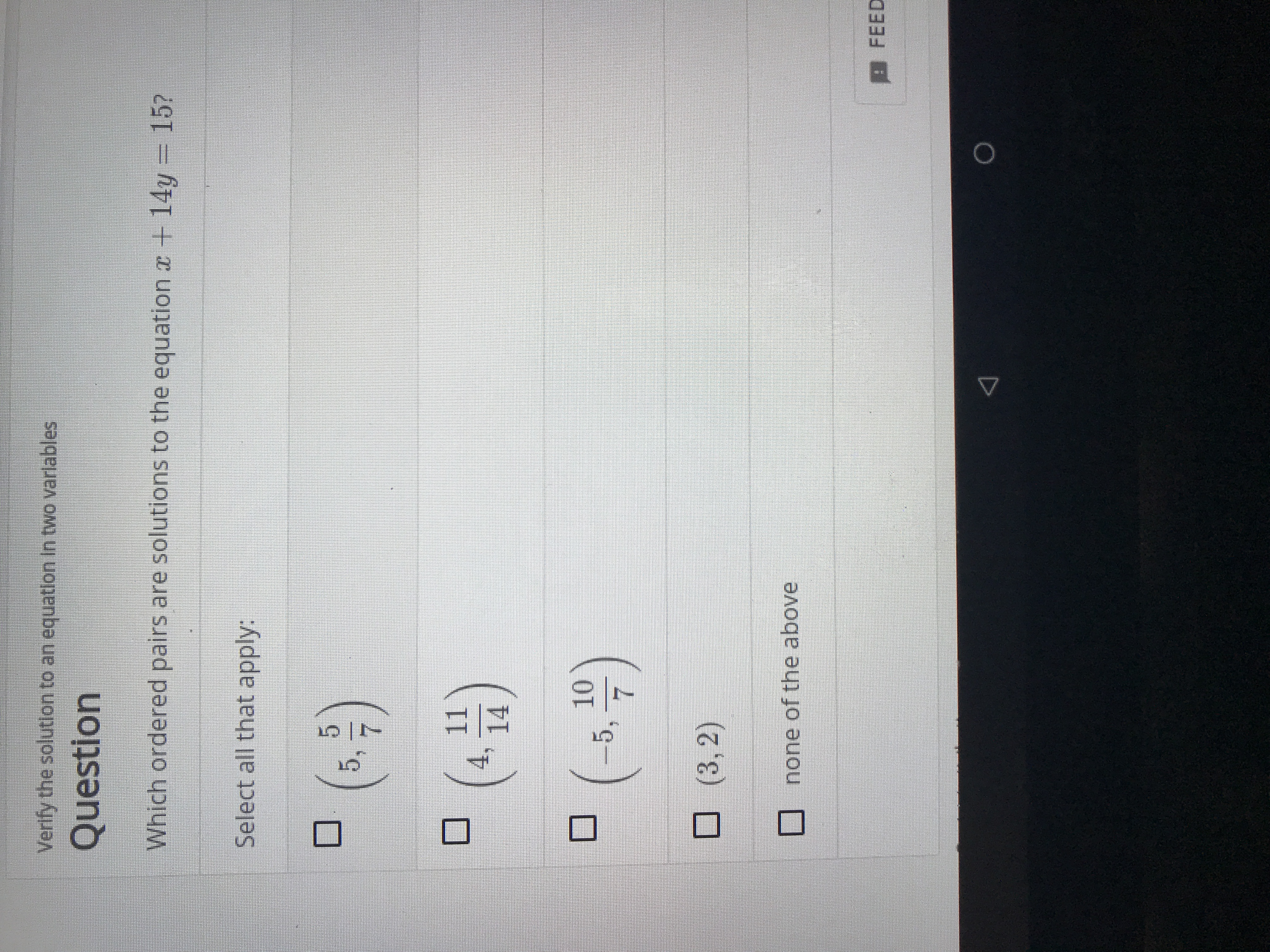# Verlfy the solution to an equation In two varlables Question Which ordered pairs are solutions to the equation r +14y= 15? Select all that apply: (. 5 5, 11 4, 14 10 5, 7 (3, 2) none of the above BFEED

Questionhelp_outlineImage TranscriptioncloseVerlfy the solution to an equation In two varlables Question Which ordered pairs are solutions to the equation r +14y= 15? Select all that apply: (. 5 5, 11 4, 14 10 5, 7 (3, 2) none of the above BFEED fullscreen

### Want to see this answer and more?

Experts are waiting 24/7 to provide step-by-step solutions in as fast as 30 minutes!*